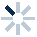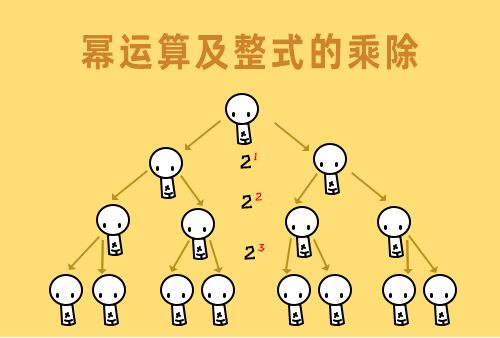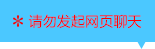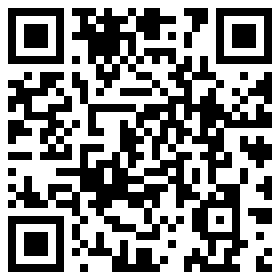| | |

||
APP端下载AndroidiPhone
|
￥40

|
2189人点赞
216625人已学习
|

3天无理由退款

3天无理由退款：退款将以超级币形式退至您的超级课堂学习账户，便于您重新选购其他课程。恶意退款将被冻结账号。

• 1、为了确定一个平面内点的位置，人们发明了平面直角坐标系。就是有公共原点而且互相垂直的两条数轴。平面直角坐标系的三个特征：两条数轴、互相垂直、原点重合
2、 如何确定坐标系内任意一点$P$的坐标：过$P$分别向$x$、$y$轴作垂线，垂足在$x$轴、$y$轴上对应的数$a$、$b$分别叫做点$P$的横坐标、纵坐标，点$P$的坐标就记作$\left ( a,b \right )$
• 1、知道$1234$象限的位置，还有每个象限内的点的坐标特征：第一象限：$\left ( +,+ \right )$第二象限：$\left ( -,+ \right )$第三象限：$\left ( -.- \right )$第四象限：$\left ( +,- \right )$
2、 坐标轴上点的坐标特征：$x$轴上的点纵坐标为$0$，记为$\left ( a,0 \right )$。$y$轴上的点横坐标为$0$，记为$\left ( 0,a \right )$，原点坐标为$\left ( 0,0 \right )$
3、 点$p \left ( a,b \right )$到$x$轴的距离为$\left | b \right |$，到$y$轴的距离为$\left | a \right |$。由此得到象限角平分线上点的坐标，分别是$\left ( a,a \right )$、$\left ( -a,a \right )$、$\left ( -a,-a \right )$和$\left ( a,-a \right )$
• 1、坐标系内点的平移规律：左右平移，横坐标左减右加；上下平移，纵坐标上加下减
2、 两个方向同时平移时只需要单独考虑横坐标和纵坐标的变化情况，两种变化互不干扰
3、 根据坐标的变化情况也可以得出平移的方向和平移量，作法是把平移规律反过来用
4、 图形的平移规律：在图形的平移中，图形中的每一个点都向相同的方向平移相同的距离。因此图形的平移问题实质上还是点的平移问题
• 1、点的坐标特征：平行于$x$轴的直线上，点纵坐标相同；平行于$y$轴的直线上，点横坐标相同
2、 是关于$x$轴、$y$轴和原点对称的两点的坐标特征：关于$x$轴对称的点，$x$坐标相同，$y$坐标互为相反数；关于$y$轴对称的点，$y$坐标相同，$x$坐标互为相反数；关于原点对称的两点横纵坐标都互为相反数
3、 对称图形的画法：根据对称的坐标规律，画出各顶点相应的对称点，再连起来就是对称图形
• 1、$AB$两点的距离公式，设$A$、$B$的坐标分别为$\left ( x_{1},y_{1} \right )$、$\left ( x_{2},y_{2} \right )$
2、 若平行于$x$轴，$A$、$B$两点纵坐标相等，$AB=\left | x_{1}-x_{2} \right |$
3、 若平行于$y$轴，$A$、$B$两点横坐标相等，$AB=\left | y_{1}-y_{2} \right |$
4、 然后是任意两点间的距离公式：根号下的$(x_{1}-x_{2})^{2}+(y_{1}-y_{2})^{2}$。需要认真体会利用勾股定理得到这个公式的思想
5、 最后，坐标为$\left ( x,y \right )$的点$A$，到原点的距离
• 平面直角坐标系综合练习

• 1马上就到你
• 2超级学员4108890
• 3超级学员4165375
• 4超级学员4197097
• 5超级学员4239069
• 6超级学员4247628
• 7超级学员4271634
• 8超级学员4290711
• 9超级学员4302927
• 10超级学员4326579217874人在学
￥ 88 ￥ 88267787人在学
￥ 12 ￥ 12216522人在学
￥ 88 ￥ 88216827人在学
￥ 63 ￥ 63

• 0
• 点击分享有好礼
••app端下载关注微信号